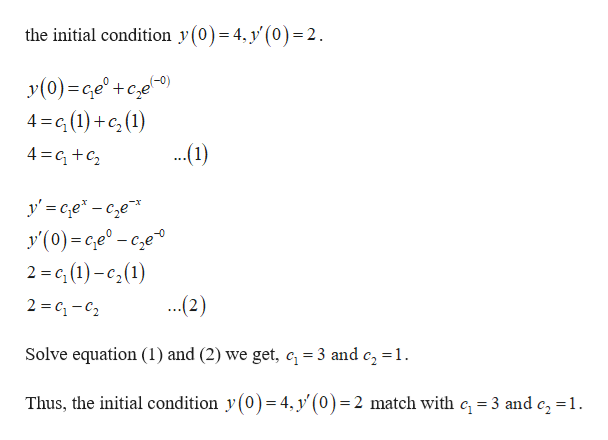# Calculus Question

Question
check_circle

Step 1

Given:

Step 2

Apply the initial condition as follows.help_outlineImage Transcriptionclosethe initial condition y(0)= 4, y' (0) = 2. y(0) e +ce) 4 G(1) (1) ...1) 4 c y Ge-ce y(0)-ce° - cze 2 = (1)-c2(1) ...2) 2 C-C2 Solve equation (1) and (2) we get, c 3 and c, =1 Thus, the initial condition y(0)= 4, y'(0)= 2 match with c, 3 and c2 =1 fullscreen
Step 3

Apply the initial condi...

### Want to see the full answer?

See Solution

#### Want to see this answer and more?

Solutions are written by subject experts who are available 24/7. Questions are typically answered within 1 hour.*

See Solution
*Response times may vary by subject and question.
Tagged in

### Derivative